# Get help with conic sections equationsRecent questions in Conic sections
Conic sections
ANSWERED### The current in a wire varies with time according to the relation $$\displaystyle{I}={55}{A}?(0.65\frac{A}{s^2}){t}^{2}$$. How many coulombs of charge pass a cross section of the wire in the time interval between $$\displaystyle{t}={0}$$ and $$\displaystyle{t}={8.5}{s}$$ ? Express your answer using two significant figures. What constant current would transport the same charge in the same time interval? Express your answer using two significant figures.

Conic sections
ANSWERED### Sketch the following conic sections: $$\displaystyle{4}^{{{2}}}-{4}{y}+{8}-{4}{x}^{{{2}}}={0}$$

Conic sections
ANSWERED### To solve the given inequality and to write the solution set in interval notation.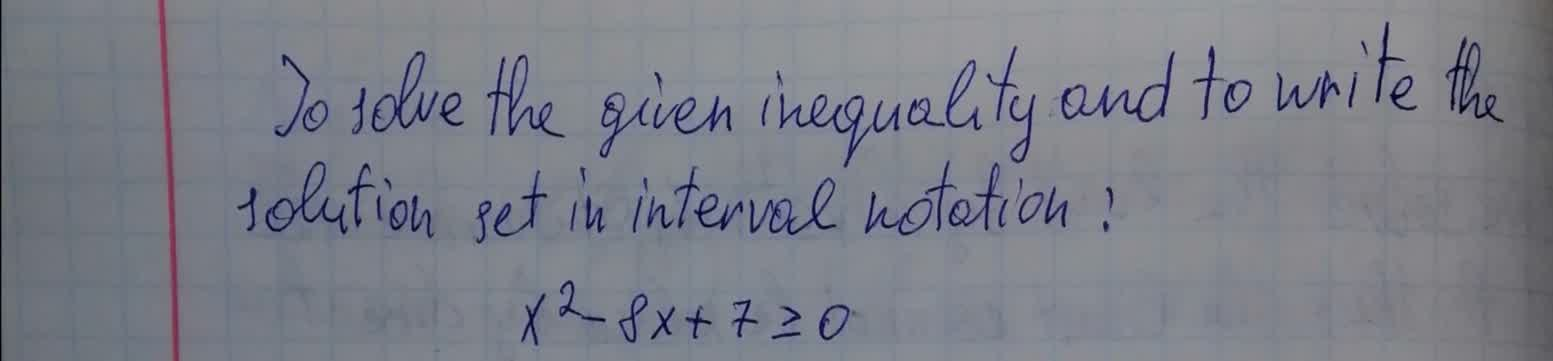Conic sections
ANSWERED### To simplify: The given radical expression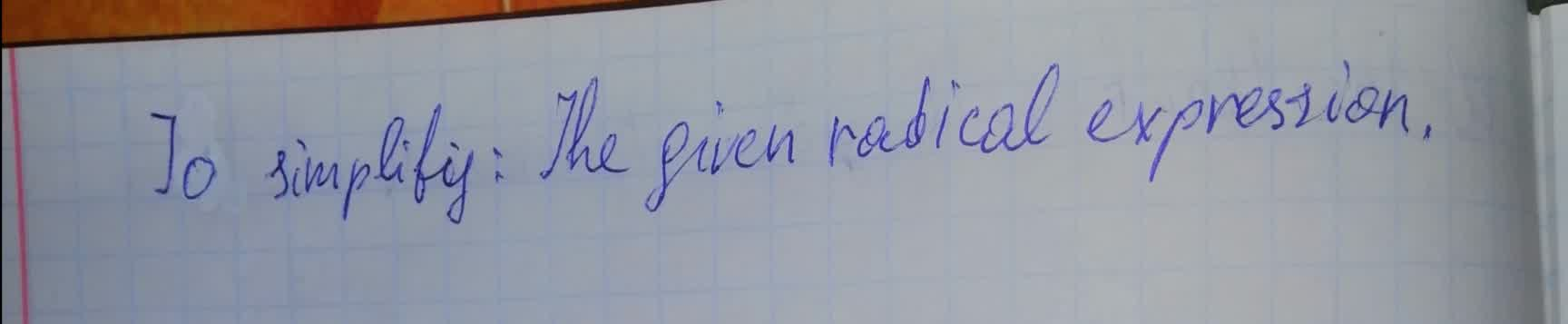Conic sections
ANSWERED### To simplify the given expression.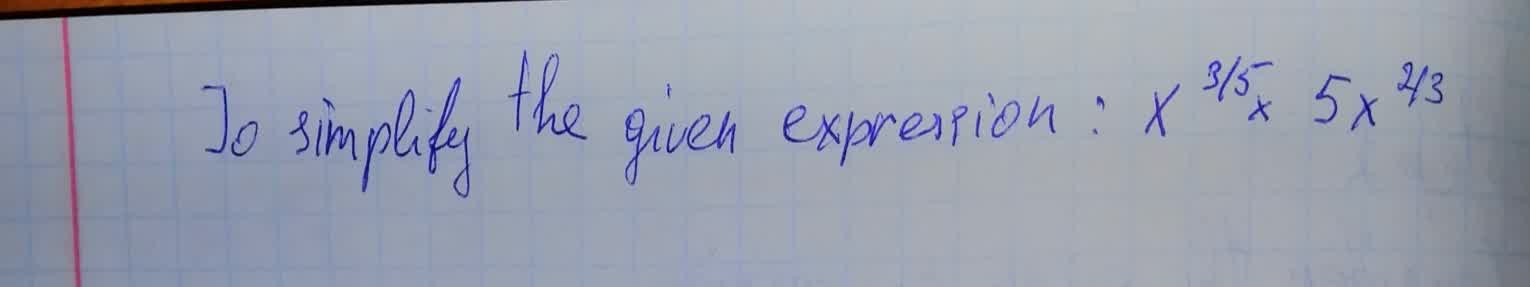Conic sections
ANSWERED### To determine: The radical form of $$\sqrt{64}$$ in the rational exponent form.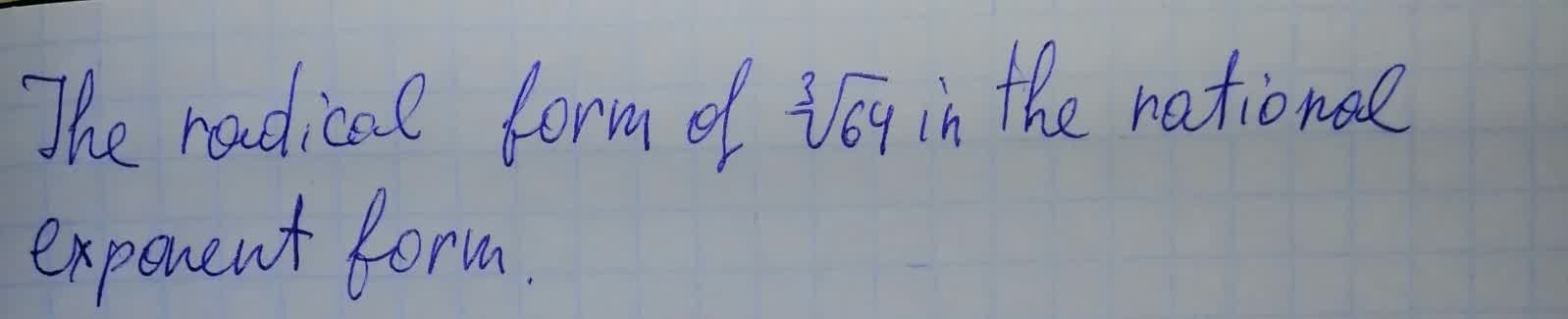Conic sections
ANSWERED### To calculate: The simplification of the expression $$\displaystyle{\frac{{{7}}}{{\sqrt{{{3}{x}}}}}}+{\frac{{\sqrt{{{3}{x}}}}}{{{x}}}}$$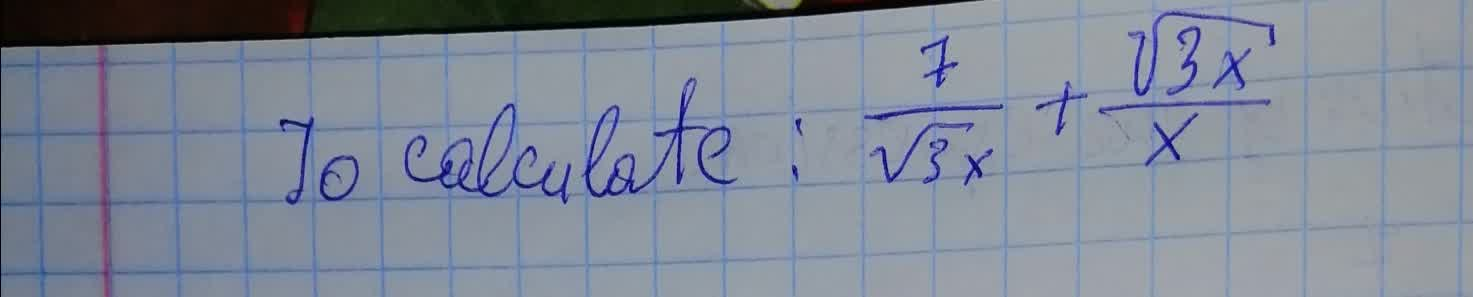Conic sections
ANSWERED### To calculate: The simplification of the expression $$\displaystyle{\frac{{\sqrt{{{50}}}}}{{\sqrt{{{x}-{2}}}}}}$$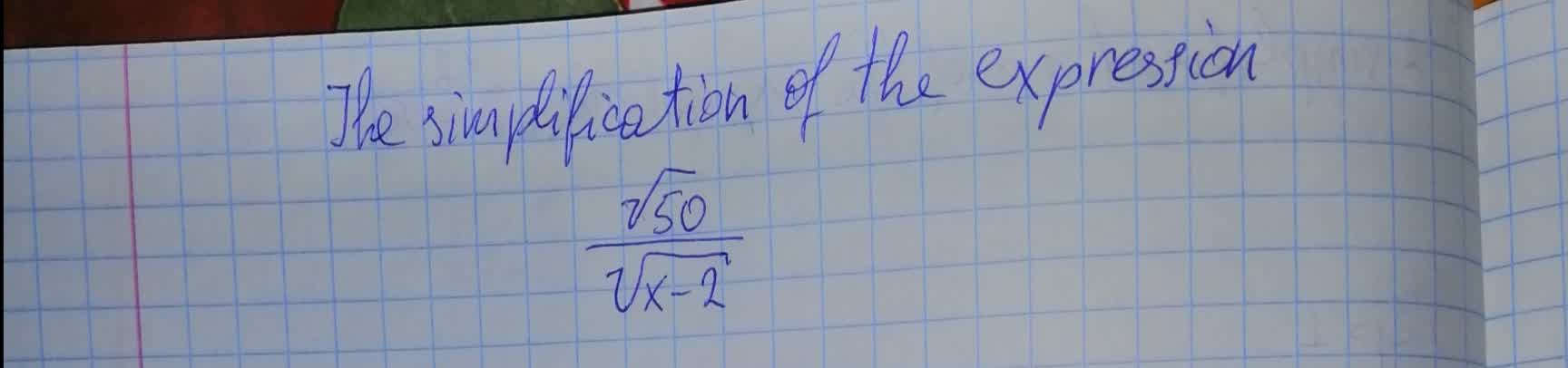Conic sections
ANSWERED### To calculate: The simplified form of the expression , $$\displaystyle{\frac{{{12}}}{{\sqrt{{{6}}}+\sqrt{{2}}}}}$$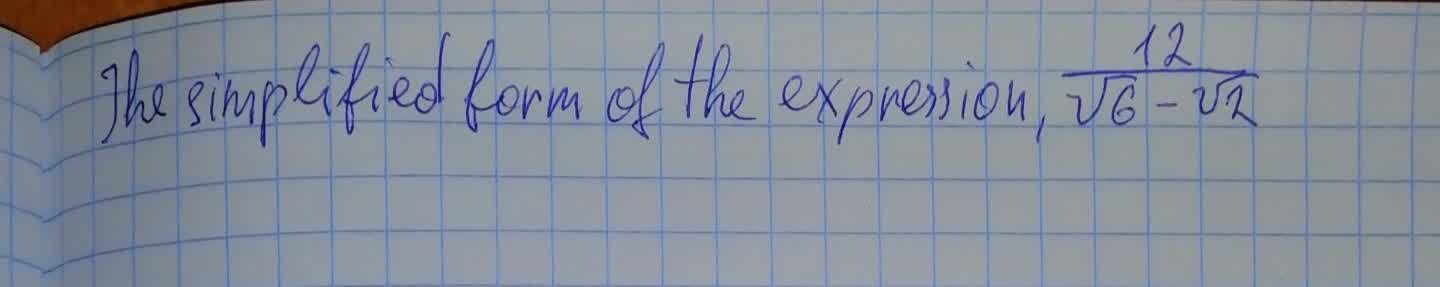Conic sections
ANSWERED### Give the eccentricities of conic sections with one focus at the origin along with the directrix corresponding to that focus. Find a polar equation for each conic section. 1) $$\displaystyle{e}={\frac{{{1}}}{{{4}}}},\ {x}=-{2}$$ 2) $$\displaystyle{e}={\frac{{{1}}}{{{3}}}},\ {y}={6}$$

Conic sections
ANSWERED### Give the eccentricities of conic sections with one focus at the origin along with the directrix corresponding to that focus. Find a polar equation for each conic section. $$\displaystyle{e}=\frac{{1}}{{5}},\ {y}=-{10}$$

Conic sections
ANSWERED### To identify: The conic represented by the following polar equation and graph it by using the graphing utility: $$\displaystyle{r}={\frac{{{8}}}{{{4}+{3}{\sin{\theta}}}}}$$

Conic sections
ANSWERED### Graph the lines and conic sections $$\displaystyle{r}={\frac{{{8}}}{{{4}+{\sin{\theta}}}}}$$

Conic sections
ANSWERED### To determine: The radical exponent form of $$\displaystyle{3}{x}^{{-{\frac{{{2}}}{{{3}}}}}}$$ in radical form.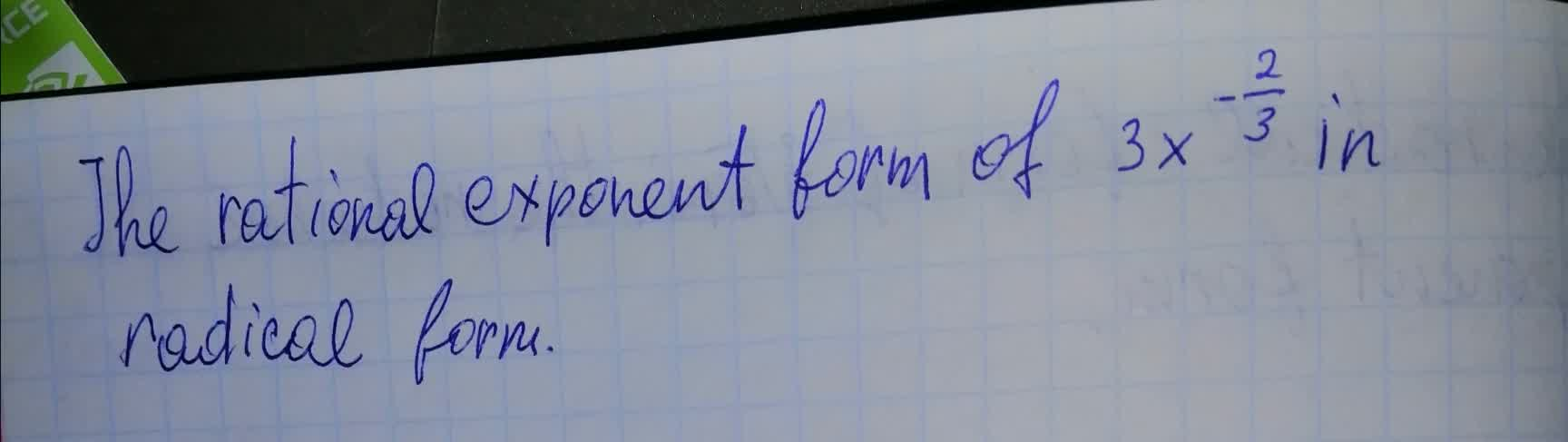Conic sections
ANSWERED### The rational exponent form of $$\displaystyle{a}^{{{0.4}}}$$ in radical form.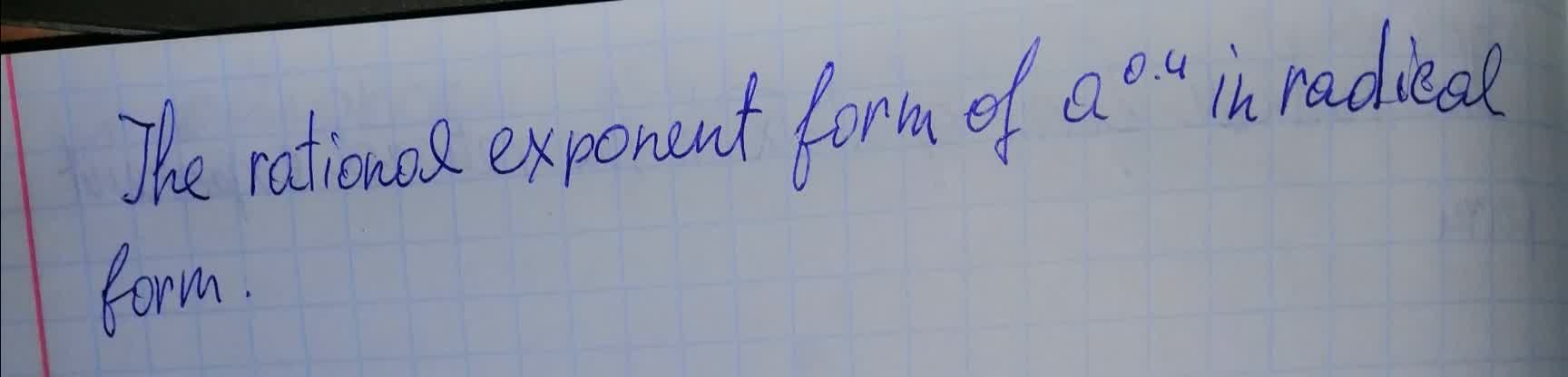Conic sections
ANSWERED### To calculate: The simplified form of the expression , $$\displaystyle\sqrt{{{\frac{{{1.44}\times{10}^{{{16}}}}}{{{9.0}\times{10}^{{{10}}}}}}}}$$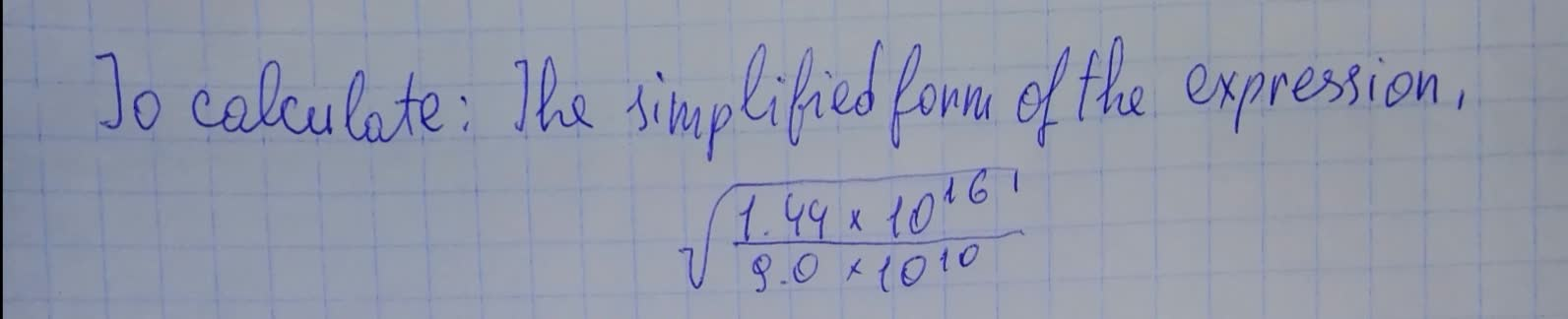Conic sections
ANSWERED### To calculate : The domain of the expression $$\displaystyle\sqrt{{{x}-{2}}}$$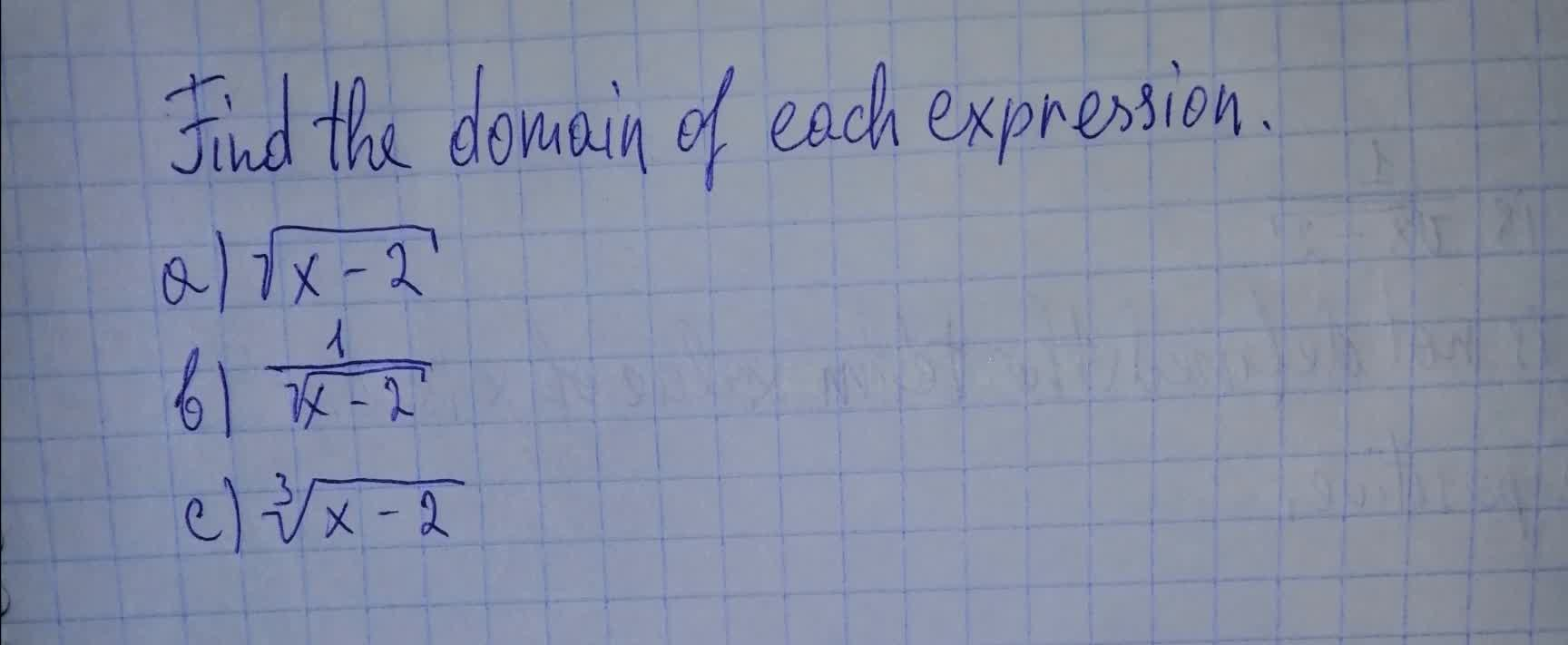Conic sections
ANSWERED### To calculate: The simplification of the expression $$\displaystyle{\frac{{{y}-{3}}}{{\sqrt{{{y}}}+\sqrt{{{3}}}}}}$$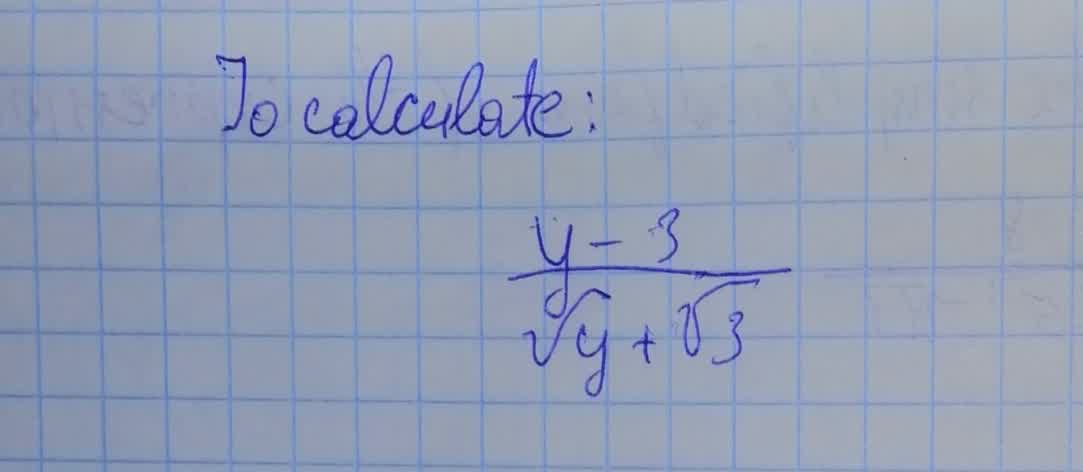Conic sections
ANSWERED### To calculate: The simplification of the expression $$\frac{7}{\sqrt{z}}$$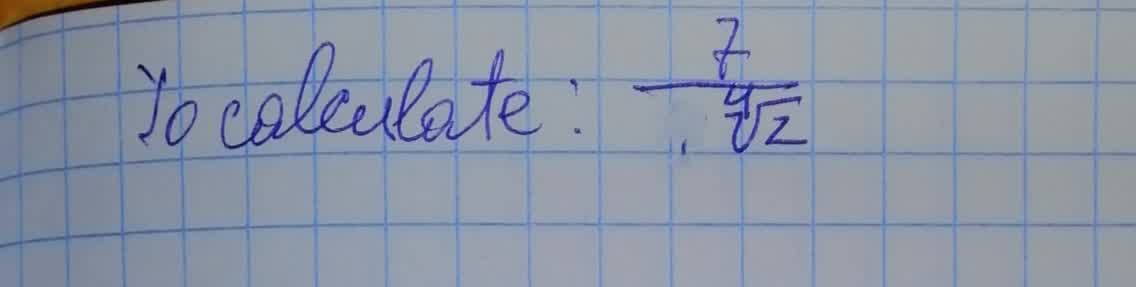ANSWERED